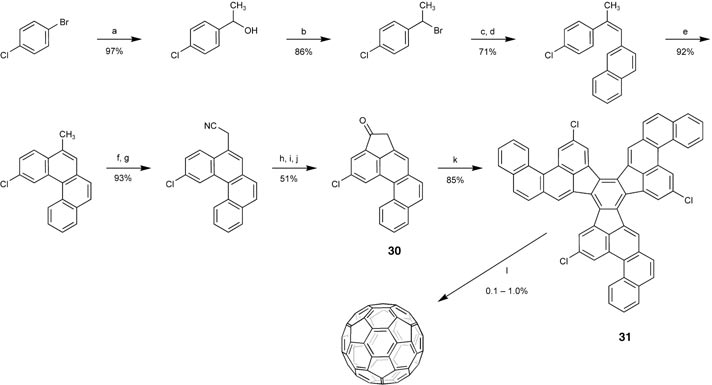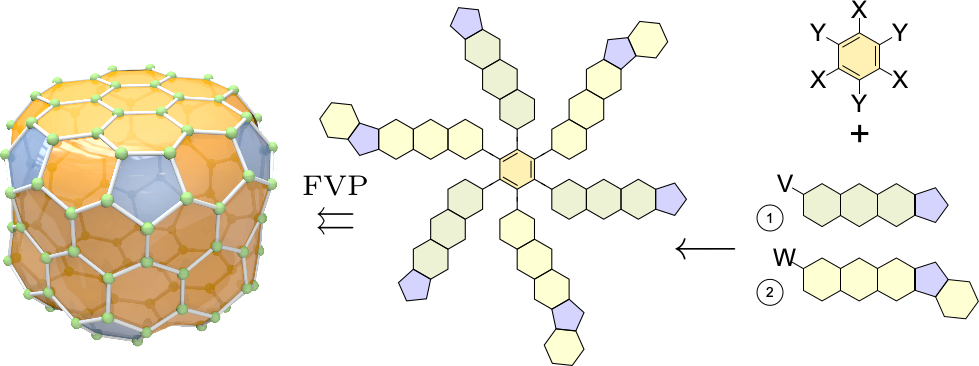## Background

Only a small handful of fullerenes have so far been realized experimentally. These are produced at several thousand Kelvin by vaporization of graphite followed by chromotography of the resulting soot, or by combustion of simple hydrocarbons in fuel-rich flames. While this can produce I$_h$-C$_{60}$ in kilogram quantities, it always produces the same few fullerenes, mainly I$_h$-C$_{60}$ and D$_{5h}$-C$_{70}$. There is little hope that specific fullerene structures found to have interesting properties can be produced by such methods. Gaining access to the full space of fullerene isomers will require methods for direct chemical synthesis.

Scott et al. pioneered rational synthesis of fullerenes (and geodesic polyarenes in general), successfully synthesizing I$_h$-C$_{60}$ directly by constructing, in a sequence of high-yield steps, a planar precursor that autoassembled to I$_h$-C$_{60}$ in flash vacuum pyrolysis (Scott et al. 2002, Scott et al. 2004). While yields of the final FVP stage were low (0.1-1%) because of the harsh reaction conditions, Otero et al. devised a highly efficient surface-catalysed cyclodehydrogenation process that improved the autoassembly stage to nearly 100% yield (Otero et al. 2008); and in Kabdulov et al. 2010, the precursor was improved with fluorine as radical promoter, which could be introduced in the most sterically impeded regions at key positions to control the folding stage.Fig.1: Rational synthesis for $I_h$-C$_{60}$ (Scott 2004). Radical-controlled cage autoassembly through flash vacuum pyrolysis.

The planar precursor excellently matches the unfoldings to the Eisenstein plane. It is in fact a particular such unfolding with extra constraints: it should be symmetric (to allow parallel construction), the symmetric generators should correspond to stable molecules, and it must be able to fold up'' in a controlled manner, timed by radical-initiated aryl-aryl coupling reactions. Any success in exploiting this connection to aid in the discovery of precursors and retrosynthesis of arbitrary fullerenes would be groundbreaking. Task R below deals specifically with this.Fig.2: Rational synthesis precursor for a drum-shaped $D_6$-C$_{120}$ fullerene found using my prototype.

## Task R: Computing Precursors and Recipes for Rational Synthesis

In this task, we will exploit the relationship between the unfoldings and planar fullerene precursors. The goal is to build software that, given an individual fullerene isomer, aids in finding plausible precursors for its direct chemical synthesis. Subtasks include:

1. Generate precursors using maximally symmetric unfoldings with geometric constraints determined by autoassembly requirements.
2. Geometric analysis of folding and optimal placement of halogens (for low-temperature generation of radical sites and controlling folding reaction sequence).
3. Systematize building-block reactions.
4. Compute suggestions for reaction pathways by decomposition to chemically accessible fragments through a selection of synthetically feasible cuts of the carbon skeleton.

A fast but very approximate scheme can be used for screening, and a more exhaustive but slow scheme for aiding retrosynthesis of specific interesting fullerenes.

Scott's successful scheme for rational synthesis of I$_h$-C$_{60}$ was the culmination of decades of work, and there will be a great deal of know-how that must be incorporated into such a scheme.

## Preliminary Work:

I have written a prototype that computes the symmetry group in $\mathcal{O}(N)$ time (microseconds per vertex) using our generalized spirals for polyhedral representation by L. Wirz and myself (Wirz et al. 2018) and generates maximally symmetric unfoldings by a variant of Fontet's algorithm (Eades and Hong 2013, Chapter 3).

The central code of the prototype is listed in Fig. 4. An example precursor for a D$_6$-C$_{120}$ is shown in Fig. 2, constructed using this code in Fig. 3. The final decomposition was done by hand together with Prof. Laursen. A part of Task R will be to formalize and automate this step. The symmetry group and point group information is computed as described here. A maximal planar subgroup of the full point group is computed using the method in Fig. 3.